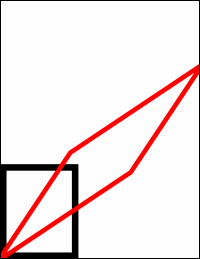Skew MethodSkews horizontally and vertically about a locked anchor point.Syntax

 `XTransform.Skew SkewX, SkewY, AnchorX, AnchorY`Params

 Name Type Description SkewX Double The amount of horizontal skewing to apply. SkewY Double The amount of vertical skewing to apply. AnchorX Double The horizontal coordinate about which the stretch should be applied. AnchorY Double The vertical coordinate about which the stretch should be applied.Notes

 This method skews the world space about a locked anchor point. Different degrees of horizontal and vertical stretch can be used. A skew or shear is a mathematical operation which shifts points by an amount proportional to the distance from the anchor point. This shift is scaled by the horizontal and vertical skew factors.Example

 Here, we draw two rectangles into our document. The black rectangle is drawn before the skew operation, and the red one is drawn after it. ```Set theDoc = Server.CreateObject("ABCpdf12.Doc") theDoc.Rect.Width = 200 theDoc.Rect.Height = 250 theDoc.Rect.Position 20, 20 theDoc.Width = 20 theDoc.FrameRect theDoc.Transform.Skew 1.5, 1.5, 20, 20 theDoc.Color = "255 0 0" ' red theDoc.FrameRect theDoc.Save "c:\mypdfs\transformskew.pdf"```transformskew.pdf